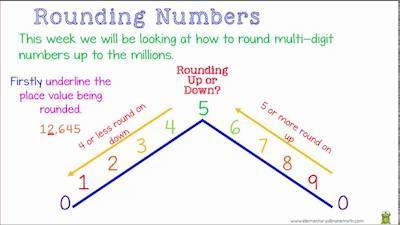# Unexpected Results When Rounding Numbers

Simply put, rounding numbers is no science. After all, we do it all the time even when we are not really aware of it. However, when you’re using a software to help you round numbers, you may need to deal with some unexpected results.

In case you have been using the SPSS Statistics software, you may have already noticed that this software sometimes doesn’t produce correct results when rounding numbers using the RND function. In fact, some of the results you get may actually be different depending on the version and release that you are using.

Discover how to easily round numbers using our calculators.

But this is not the only problem with this specific software. Sometimes, when you compute values in two different ways that are mathematically equivalent, you may get slightly different results.

Finally, you may have already noticed that if you type a number with more than 16 digits in it into a cell in the Data Editor, the value is sometimes changed by the program. Why are these things happening, and what can you do about them?

## Unexpected Results When Rounding Numbers – Solving The Problem

Simply put, the SPSS Statistics works with finite precision and is therefore unable to exactly represent or perform error-free calculations on the infinitely large collection of numbers known as real numbers.

Most of the time, the discrepancies between true mathematical results and what the program produces will be very small and will go unnoticed. However, special care must be taken in situations where comparisons among values that are extremely close together are required or where very large and/or small numbers are involved.

Looking for the best rounding algorithm?

The main problem is that the collection of numbers designated by mathematicians as the field of real numbers contains an infinite or unlimited number of values, while the number of physical locations available for representation or storage of a number in a computer is finite or limited.

The numbers that can be stored or represented in a computer are thus a subset of the real numbers. This subset is limited in the range of numbers represented and in terms of precision. That is, some numbers are too large in magnitude to be represented in a computer, and within the range of numbers that can be represented, there is a limit to the precision with which numbers can be represented.

This is how you multiply decimals.

While there are some types of problems that can be handled via analytical methods that produce exact results via what is known as symbolic computations, many of the types of problems routinely handled by SPSS Statistics cannot be dealt with in this way. While extended precision methods such as quadruple precision can make the errors smaller, they do not eliminate the problems and have costs in terms of memory and processing time. So-called arbitrary precision methods have prohibitive costs in terms of memory and processing time. None of these approaches represent a practical solution to these issues.

Learn how to round numbers in C and C++.

From the perspective of a user of the software, it is important to recognize that some small amounts of error are going to be involved in computations you perform with SPSS Statistics and similar programs. In the vast majority of situations, you are unlikely to notice these errors and they are benign, with no practical effects on your results. The situations in which you generally need to be careful are those in which operations are performed that rely on equality comparisons, such as rounding and truncation.